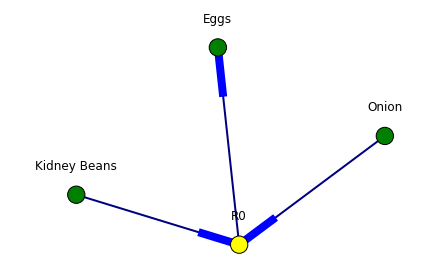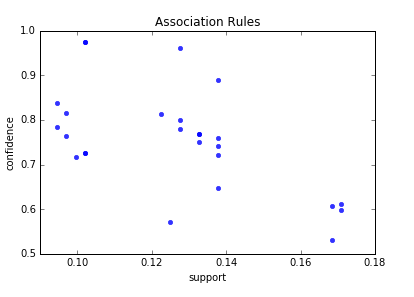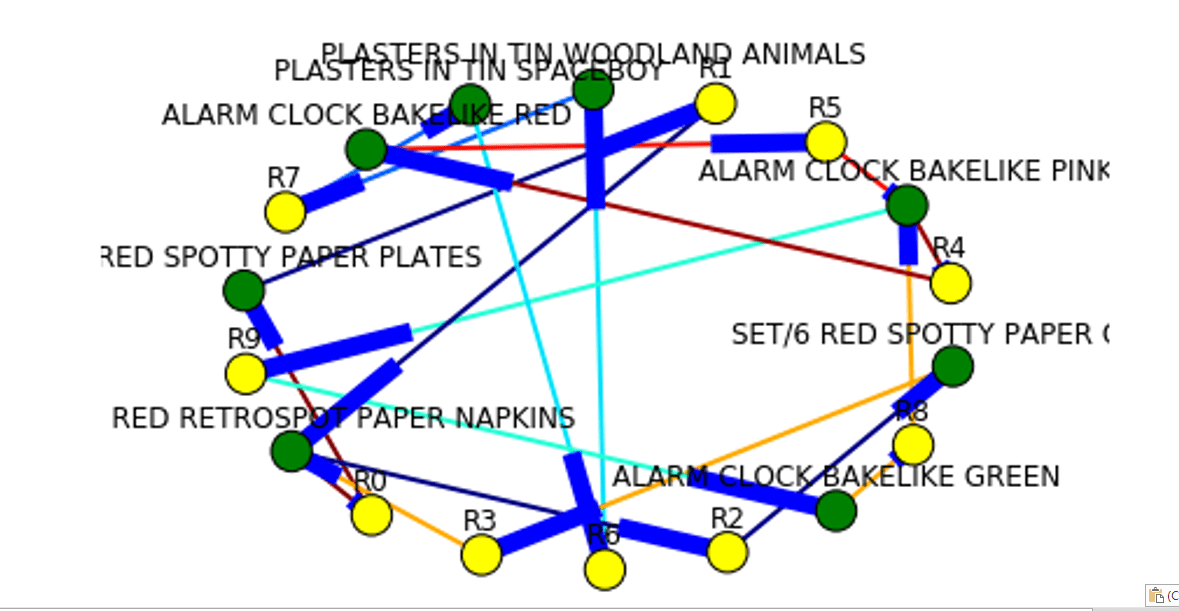## How to Create Data Visualization for Association Rules in Data Mining

Association rule learning is used in machine learning for discovering interesting relations between variables. Apriori algorithm is a popular algorithm for association rules mining and extracting frequent itemsets with applications in association rule learning. It has been designed to operate on databases containing transactions, such as purchases by customers of a store (market basket analysis).  Besides market basket analysis this algorithm can be applied to other problems. For example in web user navigation domain we can search for rules like customer who visited web page A and page B also visited page C.

Python sklearn library does not have Apriori algorithm but recently I come across post  where python library MLxtend was used for Market Basket Analysis. MLxtend has modules for different tasks. In this post I will share how to create data visualization for association rules in data mining using MLxtend for getting association rules and NetworkX module for charting the diagram. First we need to get association rules.

### Getting Association Rules from Array Data

To get association rules you can run the following code

```dataset = [['Milk', 'Onion', 'Nutmeg', 'Kidney Beans', 'Eggs', 'Yogurt'],
['Dill', 'Onion', 'Nutmeg', 'Kidney Beans', 'Eggs', 'Yogurt'],
['Milk', 'Apple', 'Kidney Beans', 'Eggs'],
['Milk', 'Unicorn', 'Corn', 'Kidney Beans', 'Yogurt'],
['Corn', 'Onion', 'Onion', 'Kidney Beans', 'Ice cream', 'Eggs']]

import pandas as pd
from mlxtend.preprocessing import OnehotTransactions
from mlxtend.frequent_patterns import apriori

oht = OnehotTransactions()
oht_ary = oht.fit(dataset).transform(dataset)
df = pd.DataFrame(oht_ary, columns=oht.columns_)
print (df)

frequent_itemsets = apriori(df, min_support=0.6, use_colnames=True)
print (frequent_itemsets)

association_rules(frequent_itemsets, metric="confidence", min_threshold=0.7)
rules = association_rules(frequent_itemsets, metric="lift", min_threshold=1.2)
print (rules)

"""
Below is the output
support                     itemsets
0       0.8                       [Eggs]
1       1.0               [Kidney Beans]
2       0.6                       [Milk]
3       0.6                      [Onion]
4       0.6                     [Yogurt]
5       0.8         [Eggs, Kidney Beans]
6       0.6                [Eggs, Onion]
7       0.6         [Kidney Beans, Milk]
8       0.6        [Kidney Beans, Onion]
9       0.6       [Kidney Beans, Yogurt]
10      0.6  [Eggs, Kidney Beans, Onion]

antecedants            consequents  support  confidence  lift
0  (Kidney Beans, Onion)                 (Eggs)      0.6        1.00  1.25
1   (Kidney Beans, Eggs)                (Onion)      0.8        0.75  1.25
2                (Onion)   (Kidney Beans, Eggs)      0.6        1.00  1.25
3                 (Eggs)  (Kidney Beans, Onion)      0.8        0.75  1.25
4                (Onion)                 (Eggs)      0.6        1.00  1.25
5                 (Eggs)                (Onion)      0.8        0.75  1.25

"""
```

### Confidence and Support in Data Mining

To select interesting rules we can use best-known constraints which are a minimum thresholds on confidence and support.
Support is an indication of how frequently the itemset appears in the dataset.
Confidence is an indication of how often the rule has been found to be true. 

```support=rules.as_matrix(columns=['support'])
confidence=rules.as_matrix(columns=['confidence'])
```

Below is the scatter plot for support and confidence:

And here is the python code to build scatter plot. Since few points here have the same values I added small random values to show all points.

```import random
import matplotlib.pyplot as plt

for i in range (len(support)):
support[i] = support[i] + 0.0025 * (random.randint(1,10) - 5)
confidence[i] = confidence[i] + 0.0025 * (random.randint(1,10) - 5)

plt.scatter(support, confidence,   alpha=0.5, marker="*")
plt.xlabel('support')
plt.ylabel('confidence')
plt.show()
```

### How to Create Data Visualization with NetworkX for Association Rules in Data Mining

To represent association rules as diagram, NetworkX python library is utilized in this post. Here is the association rule example :
(Kidney Beans, Onion) ==> (Eggs)

Directed graph below is built for this rule and shown below. Arrows are drawn as just thicker blue stubs. The node with R0 identifies one rule, and it will have always incoming and outcoming edges. Incoming edge(s) will represent antecedants and the stub (arrow) will be next to node.Below is the example of graph for all rules extracted from example dataset.Here is the source code to build association rules with NetworkX. To call function use draw_graph(rules, 6)

```def draw_graph(rules, rules_to_show):
import networkx as nx
G1 = nx.DiGraph()

color_map=[]
N = 50
colors = np.random.rand(N)
strs=['R0', 'R1', 'R2', 'R3', 'R4', 'R5', 'R6', 'R7', 'R8', 'R9', 'R10', 'R11']

for i in range (rules_to_show):

for a in rules.iloc[i]['antecedants']:

G1.add_edge(a, "R"+str(i), color=colors[i] , weight = 2)

for c in rules.iloc[i]['consequents']:

for node in G1:
found_a_string = False
for item in strs:
if node==item:
found_a_string = True
if found_a_string:
color_map.append('yellow')
else:
color_map.append('green')

edges = G1.edges()
colors = [G1[u][v]['color'] for u,v in edges]
weights = [G1[u][v]['weight'] for u,v in edges]

pos = nx.spring_layout(G1, k=16, scale=1)
nx.draw(G1, pos, edges=edges, node_color = color_map, edge_color=colors, width=weights, font_size=16, with_labels=False)

for p in pos:  # raise text positions
pos[p] += 0.07
nx.draw_networkx_labels(G1, pos)
plt.show()
```

### Data Visualization for Online Retail Data Set

To get real feeling and testing on visualization we can take available online retail store dataset and apply the code for association rules graph. For downloading retail data and formatting some columns the code from  was used.

Below are the result of scatter plot for support and confidence. To build the scatter plot seaborn library was used this time. Also you can find below visualization for association rules (first 10 rules) for retail data set.Here is the python full source code for data visualization association rules in data mining.

```

dataset = [['Milk', 'Onion', 'Nutmeg', 'Kidney Beans', 'Eggs', 'Yogurt'],
['Dill', 'Onion', 'Nutmeg', 'Kidney Beans', 'Eggs', 'Yogurt'],
['Milk', 'Apple', 'Kidney Beans', 'Eggs'],
['Milk', 'Unicorn', 'Corn', 'Kidney Beans', 'Yogurt'],
['Corn', 'Onion', 'Onion', 'Kidney Beans', 'Ice cream', 'Eggs']]

import pandas as pd
from mlxtend.preprocessing import OnehotTransactions
from mlxtend.frequent_patterns import apriori

oht = OnehotTransactions()
oht_ary = oht.fit(dataset).transform(dataset)
df = pd.DataFrame(oht_ary, columns=oht.columns_)
print (df)

frequent_itemsets = apriori(df, min_support=0.6, use_colnames=True)
print (frequent_itemsets)

from mlxtend.frequent_patterns import association_rules

association_rules(frequent_itemsets, metric="confidence", min_threshold=0.7)
rules = association_rules(frequent_itemsets, metric="lift", min_threshold=1.2)
print (rules)

support=rules.as_matrix(columns=['support'])
confidence=rules.as_matrix(columns=['confidence'])

import random
import matplotlib.pyplot as plt

for i in range (len(support)):
support[i] = support[i] + 0.0025 * (random.randint(1,10) - 5)
confidence[i] = confidence[i] + 0.0025 * (random.randint(1,10) - 5)

plt.scatter(support, confidence,   alpha=0.5, marker="*")
plt.xlabel('support')
plt.ylabel('confidence')
plt.show()

import numpy as np

def draw_graph(rules, rules_to_show):
import networkx as nx
G1 = nx.DiGraph()

color_map=[]
N = 50
colors = np.random.rand(N)
strs=['R0', 'R1', 'R2', 'R3', 'R4', 'R5', 'R6', 'R7', 'R8', 'R9', 'R10', 'R11']

for i in range (rules_to_show):

for a in rules.iloc[i]['antecedants']:

G1.add_edge(a, "R"+str(i), color=colors[i] , weight = 2)

for c in rules.iloc[i]['consequents']:

for node in G1:
found_a_string = False
for item in strs:
if node==item:
found_a_string = True
if found_a_string:
color_map.append('yellow')
else:
color_map.append('green')

edges = G1.edges()
colors = [G1[u][v]['color'] for u,v in edges]
weights = [G1[u][v]['weight'] for u,v in edges]

pos = nx.spring_layout(G1, k=16, scale=1)
nx.draw(G1, pos, edges=edges, node_color = color_map, edge_color=colors, width=weights, font_size=16, with_labels=False)

for p in pos:  # raise text positions
pos[p] += 0.07
nx.draw_networkx_labels(G1, pos)
plt.show()

draw_graph (rules, 6)

df['Description'] = df['Description'].str.strip()
df.dropna(axis=0, subset=['InvoiceNo'], inplace=True)
df['InvoiceNo'] = df['InvoiceNo'].astype('str')
df = df[~df['InvoiceNo'].str.contains('C')]

.groupby(['InvoiceNo', 'Description'])['Quantity']
.sum().unstack().reset_index().fillna(0)
.set_index('InvoiceNo'))

def encode_units(x):
if x <= 0:
return 0
if x >= 1:
return 1

rules = association_rules(frequent_itemsets, metric="lift", min_threshold=1)

print (rules)

support=rules.as_matrix(columns=['support'])
confidence=rules.as_matrix(columns=['confidence'])

import seaborn as sns1

for i in range (len(support)):
support[i] = support[i]
confidence[i] = confidence[i]

plt.title('Association Rules')
plt.xlabel('support')
plt.ylabel('confidence')
sns1.regplot(x=support, y=confidence, fit_reg=False)

plt.gcf().clear()
draw_graph (rules, 10)

```

References